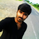37761 views
This script is based on Secret of Pivot Boss book by Frank Ochoa. Suitable for Intraday Trading.

You can view Daily timeframe cpr / support/resistance .
You can view Weekly timeframe cpr / support/resistance .
You can view Monthly timeframe cpr / support/resistance .

You can also view Previous Day High/Low.
You can also view Previous Week High/Low.
You can also view Previous Month High/Low.

You can also turn on/off as per your wish.
Protected script
This script is published closed-source and you may use it freely. You can favorite it to use it on a chart. You cannot view or modify its source code.
Want to use this script on a chart?transtechbala521ca8f6cc32426d//Created by ChrisMoody 11-23-14 with Special Thanks to TheLark...AKA...The Coding Genius
study(title="CM_Pivot Points_M-W-D_4H_1H_Filtered", shorttitle="CM_Pivots_Filtered", overlay=true)
pf = input(true,title="Show Filtered Pivots")
sa = input(true,title="Show Pivot Average")
sh = input(false, title="Show 1 Hour Pivots?")
sf = input(false, title="Show 4 Hour Pivots?")
sd = input(false, title="Show Daily Pivots?")
sw = input(false, title="Show Weekly Pivots?")
sm = input(true, title="Show Monthly Pivots?")
sy = input(false, title="Show Yearly Pivots?")
sh3 = input(false, title="Show R3 & S3 Only On 1 Hour & 4 Hour?")

// Classic Pivot
pivot = (high + low + close ) / 3.0
// Filter Cr
bull= pivot > (pivot + pivot) / 2 + .0025
bear= pivot < (pivot + pivot) / 2 - .0025
// Classic Pivots
r1 = pf and bear ? pivot + (pivot - low) : pf and bull ? pivot + (high - low) : pivot + (pivot - low)
s1 = pf and bull ? pivot - (high - pivot) : pf and bear ? pivot - (high - low) : pivot - (high - pivot)
r2 = pf ? na : pivot + (high - low)
s2 = pf ? na : pivot - (high - low)
r3 = sh3 and r1 + (high - low) ? r1 + (high - low) : na
s3 = sh3 and s1 - (high - low) ? s1 - (high - low) : na
//Pivot Average Calculation
smaP = sma(pivot, 3)

//1 Hour Pivots
htime_pivot = security(tickerid, '60', pivot)
htime_pivotAvg = security(tickerid, '60', smaP)
htime_R1 = security(tickerid, '60', r1)
htime_S1 = security(tickerid, '60', s1)
htime_R2 = security(tickerid, '60', r2)
htime_S2 = security(tickerid, '60', s2)
htime_R3 = security(tickerid, '60', r3)
htime_S3 = security(tickerid, '60', s3)

plot(sa and sh and htime_pivotAvg ? htime_pivotAvg : na, title="Hourly Pivot Average",style=cross, color=orange,linewidth=2)
plot(sh and htime_pivot ? htime_pivot : na, title="Hourly Pivot",style=circles, color=fuchsia,linewidth=2)
plot(sh and htime_R1 ? htime_R1 : na, title="Hourly R1",style=circles, color=#DC143C,linewidth=2)
plot(sh and htime_S1 ? htime_S1 : na, title="Hourly S1",style=circles, color=lime,linewidth=2)
plot(sh and htime_R2 ? htime_R2 : na, title="Hourly R2",style=circles, color=maroon,linewidth=2)
plot(sh and htime_S2 ? htime_S2 : na, title="Hourly S2",style=circles, color=#228B22,linewidth=2)
plot(sh and htime_R3 ? htime_R3 : na, title="Hourly R3",style=circles, color=#FA8072,linewidth=2)
plot(sh and htime_S3 ? htime_S3 : na, title="Hourly S3",style=circles, color=#CD5C5C,linewidth=2)

//4 Hour Pivots
ftime_pivot = security(tickerid, '240', pivot)
ftime_pivotAvg = security(tickerid, '240', smaP)
ftime_R1 = security(tickerid, '240', r1)
ftime_S1 = security(tickerid, '240', s1)
ftime_R2 = security(tickerid, '240', r2)
ftime_S2 = security(tickerid, '240', s2)
ftime_R3 = security(tickerid, '240', r3)
ftime_S3 = security(tickerid, '240', s3)

plot(sa and sf and ftime_pivotAvg ? ftime_pivotAvg : na, title="4 Hour Pivot Average",style=cross, color=orange,linewidth=2)
plot(sf and ftime_pivot ? ftime_pivot : na, title="4 Ho